﻿ Logical Symmetries and Complex-Valued Logic

## Logical Symmetries and Complex-Valued Logic

Traditionally, the algebraic formulations of logic would represent the logical value true with the number 1, and the logical value false with the number 0. Then the operations of conjunction (Ù) and disjunction (Ú) get naturally represented by algebraic multiplication and addition respectively. The rules of thus defined algebra significantly differ from common arithmetic; still, operating with numbers rather than special logical values makes certain aspects of logical theory more transparent, allowing for many productive generalizations.

There is a different approach that might sometimes come handy in logical development. Instead of representing the logical value false with 0, one could rather represent it with the number –1. Denoting the logical negation with the minus sign, we can naturally obtain –1 (false) as not true, while –(–1) equals 1 by definition: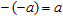,

with the letters like a, b, c, ... denoting, by convention, anything evaluating to a logical value: a constant, a variable, or a logical expression (formula) containing constants, variables and other expressions in any combination; further, one can consider logical-valued functions as abbreviations for some kinds of expressions and move on to variable functions and functional formulas; for our algebraic consideration this hierarchy is not really important.

Now, let us introduce algebraic multiplication * so that 1 * 1 = 1 and (–1) * (–1) = 1, with the natural choice of (–1) * 1 = 1 * (–1) = –1. This operation obviously corresponds to logical equivalence, and it is commutative and associative: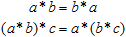but, unlike conjunction and disjunction, it is not idempotent: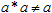which does not seem to make much trouble, since the common numeric multiplication is not idempotent either. One can also observe that,

which pleasingly complies with our the arithmetic habits.

Thus introduced multiplication is symmetrical in respect to truth valuation: swapping the truth values 1 ↔ (–1) will produce the same valuation table for logical equivalence.

It should be stressed that the equality sign in the above expressions, albeit a kind of equivalence too, belongs to the logic of our description (methodological level) rather than to the logical structures to describe. The two levels may differ in their algebraic structure, including the very specification of the logical values. We may use equality and inequality to compare the formulas of the logic in question, but not within any logical formula.

Using the above equations, we can formally eliminate negation from our theory replacing it with multiplication: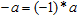.

This opens a promising direction of thought, since logical negation is a very specific operation that has historically raised much controversy. It is not quite obvious why we should stick to it in multi-valued or fuzzy logics; all we can refer to is a long-lived tradition. However, as one can see, negation is not entirely eliminated in this approach, since we have to prefer one logical value to another; this is just another way to say the same. Alternatively, one could treat non-equivalence as an independent binary operation: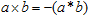,

which implies the introduction of the "complementary negation":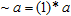and thus a completely balanced theory with no unary operations. The applications of such a logic are to stress the practical polarity of the activities establishing equivalence and those that seek for distinction, with a wide range of intermediate modes.

Obviously, the truth-values of this symmetrized logic are expressed through the two basic operations: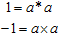for any a. However, since this definition implicitly introduces a quantor, it belongs to a higher level of hierarchy, and we cannot employ the two logical values in our algebraic logic unless we do it in a symmetrical manner, like in the example of complementary negations. In other words, the space of truth-values is thus understood as global in respect to the object area: there may be many logical values, but each of them would represent a statement about the whole of it. Still, when we mean some particular statements about the object area, we can expand the two constants as above, thus restricting ourselves to the common two-valued logic.

A fully symmetrical logic could be enriched by the introduction of asymmetric operations that make difference between falsity and truth, so that their valuation tables would no longer be the same after replacing falsity with truth, and vice versa. The two common examples are provided by conjunction and disjunction, which are interrelated through either equivalence or negation: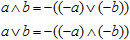One could treat these dual operations as "additive", compared to the multiplicative nature of equivalence and non-equivalence. In a logic without negation, they become independent, just like equivalence and non-equivalence. Still, in the binary case (that is, with implicit negation), we can symmetrically rewrite them as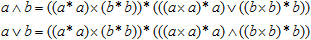or in any other similar form. Additive (asymmetric) operations might be considered as more fundamental, compared to equivalence and non-equivalence, since one could construct symmetrical combinations of asymmetric operations, but not the other way round: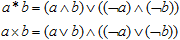Hence, the formalism of binary logic can be constructed from just two operations: logical negation and some asymmetric ("additive") operation. However, this simplicity is delusive, as it is entirely based on the idea of logical negation, to compensate one asymmetry with another. In some cases, this original constraint may get too restrictive.

Seeking for generalizations, let us recollect Peano's Arithmetices Principia. Leaving aside the artificiality of his set-theoretic formulations, we stress, that natural numbers start with postulating the existence of a unit, so that all the other natural numbers could be expressed in terms of this primary quantity. Modern mathematicians are inclined to treat the pseudo-number 0 as a natural number, which breaks the theory's transparence and consistency. Yet another important issue concerns Peano's introduction of numerical equality; later authors have identified it with logical equivalence, which is certainly inconsistent, confusing quite different levels of hierarchy just because they apparently "isomorphic". Natural arithmetic could then be derived from the unity, equality, and a single unary operation, increment (which, in general, is not the same as binary addition of a natural number and unity). However, this inductive construct did not immediately reveal the inner symmetries of the theory, and the definitions of addition and multiplication were introduced, which then lead to the notions of subtraction and division alien to the natural domain. Subtraction lead to the idea of negative numbers and positioned zero as an integer. Division produced rational arithmetic as a basis of real numbers. The same development is also possible in logic. However, here, we are interested in yet another extension, complex-valued logic.

We recall that the space of logical values in the binary limit is structured so that (–1) * (–1) = 1. Now, let us similarly introduce a logical value that would represent the square root of logical falsity: i * i = –1. Well, we do not yet know what it really is and how it can be produced, but we can simply postulate its existence and denote it with the character i. The possibility of such abstract conceptualizations has once become the cornerstone of all the modern mathematics. However, in our algebraic logic this imaginary unit value has a quite straightforward interpretation. Indeed, the equality a * a = 1 means that every real logical entity is equivalent to itself, that is, a ≡ a is true (where we mean the object area equivalence rather than the logic of its description). The equality i * i = –1 will hence introduce an entity that is not identical to itself. However strange this may sound for a scientifically minded person, the idea is not entirely new; it has long since been speculated upon in philosophy (especially in Hegel's system and in Marxism). That is, our algebraic formulation of the classical logic might become a good starting point for the reconciliation of the scientific and philosophical ways of thought provided complex truth-values are taken into account.

In this extended logic, every truth-value may contain both a real and imaginary component. Depending on the choice of the "additive" operations, one will obtain different rules for arithmetic manipulations with complex truth-values. In general, these rules will be much more complicated than in conventional complex arithmetic. This does not deny the correspondence in principle. For instance, the traditional interpretation of phase as vector rotation angle immediately applies to logical negation interpreted as vector inversion. This also leads to the distinction of inversion and mirror reflection, revealing some fine details of logicality.

The presence of the "phase" component in any logical value opens new perspectives for quantum logic, since the "observable" reasoning is restricted to real numbers, hiding the inner phase-dependent derivations. The introduction of such hidden logical states is similar to the transition from the observable position and momentum of a classical particle to the virtual motion in the inner configuration space of a quantum system that do not directly correspond to any observable quantities.

To conclude, the symmetric form of algebraic logic is promising enough to suggest a closer look to its generalized versions, including many-valued, rational, fuzzy and complex logic. It becomes especially appealing as, on the one hand, the kind of mathematics thus obtained does not demand a revolutionary shift of paradigm, and on the other hand, the new notions remain quite intuitive and tractable even for those who do not praise much the excess of formal sophistry. The hierarchy of logic has yet much room for nontrivial development.

Nov 2003